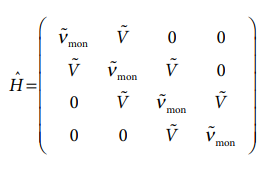×
Get Full Access to Atkins' Physical Chemistry - 11 Edition - Chapter 15g - Problem P15g.2
Get Full Access to Atkins' Physical Chemistry - 11 Edition - Chapter 15g - Problem P15g.2

×ISBN: 9780198769866 2042

## Solution for problem P15G.2 Chapter 15G

Atkins' Physical Chemistry | 11th Edition

• Textbook Solutions
• 2901 Step-by-step solutions solved by professors and subject experts
• Get 24/7 help from StudySoup virtual teaching assistantsAtkins' Physical Chemistry | 11th Edition

4 5 1 412 Reviews
18
0
Problem P15G.2

Continues from the previous problem.

(a) Consider a dimer formed of monomers which have $$\mu_{\mathrm{mon}}=4.00 \mathrm{D}$$, $$\tilde{v}_{\mathrm{mon}}=25000 \mathrm{~cm}^{-1}$$, and r=0.50 nm. Plot a graph to show how the energies (expressed as a wavenumber) of the excited states, $$\tilde{v}_{\pm}$$ vary with the angle $$\theta$$.

(b) Now expand the treatment given above to a chain of N monomers with $$\mu_{\text {mon }}=4.00 \mathrm{D}, \tilde{v}_{\text {mon }}=25000 \mathrm{~cm}^{-1}$$, and r = 0.50 nm. For simplicity, assume that $$\theta=0$$ and that only nearest neighbours interact with interaction energy $$\tilde{V}$$ (expressed here as a wavenumber). For example the hamiltonian matrix for the case N = 4 isThis matrix is analogous to the one that characterizes a band in a solid (Section 15C.1b) and so the eigenvalues (which in this case are the wavenumber of the transitions) can be written down by analogy with eqn 15C.1. Calculate the wavenumber of the lowest energy transition for N = 5, 10, and 15, and then generalize your result for large N.

(c) How does the transition dipole moment of the lowest energy transition vary with the size of the chain?

Text Transcription:

mu_mon=4.00 D

tilde v_mon=25000 cm^-1

tilde v_pm

theta

tilde V

Step-by-Step Solution:
Step 1 of 3

General Chemistry 1 Two elements are a binary compound. Covalent binary compounds are compounds composed of two non-metals (elements above the stair step). Alloys are a mixture of two metals (NaK). Ionic compounds are a mixture of metals and non-metals. Naming: Depends on the type of mixture. Ionic compounds do not use prefixes while covalent compounds use prefixes. One- mono Two-di Three-tri Four-tetra Five-penta Six-hexa Seven-hepta Eight-octa Nine-nona ten-deca Naming covalent compounds you write the first element out, and indicate how many with the prefix. Your second element do the same but with the second element it will end in -ide (Fluorine to Fluoride, Bromine to Bromide, Oxygen to Oxide, etc.). For example, CO = carbon monoxide CO2 = carbon dioxide P2O5 = diphosphorus pentoxide -With compounds containing hydrogen, we do not use prefixes (H2S = hydrogen sulfide) Ionic Compounds - composed of ions -ions are atoms or groups of atoms with a net charge. -simple ions = atoms with a net charge. -complex ions (polyatomic) = more than one atom with a net charge. -When we make our compounds they have ti have a neutral charge by definition. -Cations = positively charged & typically made of metals. -Anions = negatively charged & typically made of non-metals (Metals like to lose electrons while non-metals like to gain electrons.) -Cations + anions = ionic compound -Simple Ions = representative elements gain or lose electrons to have the same number of electrons as noble gases. If you look at where

Step 2 of 3

Step 3 of 3

##### ISBN: 9780198769866

This full solution covers the following key subjects: . This expansive textbook survival guide covers 327 chapters, and 1120 solutions. The answer to “?Continues from the previous problem. (a) Consider a dimer formed of monomers which have $$\mu_{\mathrm{mon}}=4.00 \mathrm{D}$$, $$\tilde{v}_{\mathrm{mon}}=25000 \mathrm{~cm}^{-1}$$, and r=0.50 nm. Plot a graph to show how the energies (expressed as a wavenumber) of the excited states, $$\tilde{v}_{\pm}$$ vary with the angle $$\theta$$. (b) Now expand the treatment given above to a chain of N monomers with $$\mu_{\text {mon }}=4.00 \mathrm{D}, \tilde{v}_{\text {mon }}=25000 \mathrm{~cm}^{-1}$$, and r = 0.50 nm. For simplicity, assume that $$\theta=0$$ and that only nearest neighbours interact with interaction energy $$\tilde{V}$$ (expressed here as a wavenumber). For example the hamiltonian matrix for the case N = 4 is This matrix is analogous to the one that characterizes a band in a solid (Section 15C.1b) and so the eigenvalues (which in this case are the wavenumber of the transitions) can be written down by analogy with eqn 15C.1. Calculate the wavenumber of the lowest energy transition for N = 5, 10, and 15, and then generalize your result for large N. (c) How does the transition dipole moment of the lowest energy transition vary with the size of the chain?Text Transcription:mu_mon=4.00 Dtilde v_mon=25000 cm^-1tilde v_pmthetatilde V” is broken down into a number of easy to follow steps, and 189 words. Since the solution to P15G.2 from 15G chapter was answered, more than 207 students have viewed the full step-by-step answer. Atkins' Physical Chemistry was written by Aimee Notetaker and is associated to the ISBN: 9780198769866. The full step-by-step solution to problem: P15G.2 from chapter: 15G was answered by Aimee Notetaker, our top Chemistry solution expert on 04/25/22, 03:45PM. This textbook survival guide was created for the textbook: Atkins' Physical Chemistry, edition: 11.

## Discover and learn what students are asking

Statistics: Informed Decisions Using Data : Comparing Three or More Means (One-Way Analysis of Variance)
?True or False: To perform a one-way ANOVA, the populations do not need to be normally distributed.

Statistics: Informed Decisions Using Data : Comparing Three or More Means (One-Way Analysis of Variance)
?The variability among the sample means is called ____________ sample variability, and the variability of each sample is the sample variability.

Unlock Textbook Solution